GATE  >  Production And Industrial Engineering - PI 2012 GATE Paper (Practice Test)

# Production And Industrial Engineering - PI 2012 GATE Paper (Practice Test) - GATE

Test Description

## 65 Questions MCQ Test GATE Past Year Papers for Practice (All Branches) - Production And Industrial Engineering - PI 2012 GATE Paper (Practice Test)

Production And Industrial Engineering - PI 2012 GATE Paper (Practice Test) for GATE 2023 is part of GATE Past Year Papers for Practice (All Branches) preparation. The Production And Industrial Engineering - PI 2012 GATE Paper (Practice Test) questions and answers have been prepared according to the GATE exam syllabus.The Production And Industrial Engineering - PI 2012 GATE Paper (Practice Test) MCQs are made for GATE 2023 Exam. Find important definitions, questions, notes, meanings, examples, exercises, MCQs and online tests for Production And Industrial Engineering - PI 2012 GATE Paper (Practice Test) below.
Solutions of Production And Industrial Engineering - PI 2012 GATE Paper (Practice Test) questions in English are available as part of our GATE Past Year Papers for Practice (All Branches) for GATE & Production And Industrial Engineering - PI 2012 GATE Paper (Practice Test) solutions in Hindi for GATE Past Year Papers for Practice (All Branches) course. Download more important topics, notes, lectures and mock test series for GATE Exam by signing up for free. Attempt Production And Industrial Engineering - PI 2012 GATE Paper (Practice Test) | 65 questions in 180 minutes | Mock test for GATE preparation | Free important questions MCQ to study GATE Past Year Papers for Practice (All Branches) for GATE Exam | Download free PDF with solutions
 1 Crore+ students have signed up on EduRev. Have you?
Production And Industrial Engineering - PI 2012 GATE Paper (Practice Test) - Question 1

### Q. 1 – Q. 5 carry one mark each. Q. Choose the most appropriate alternative from the options given below to complete the following sentence: Suresh’s dog is the one ––––––––– was hurt in the stampede.

Production And Industrial Engineering - PI 2012 GATE Paper (Practice Test) - Question 2

### Choose the most appropriate alternative from the options given below to complete the following sentence: Despite several ––––––––– the mission succeeded in its attempt to resolve the conflict.

Production And Industrial Engineering - PI 2012 GATE Paper (Practice Test) - Question 3

### Choose the grammatically INCORRECT sentence:

Production And Industrial Engineering - PI 2012 GATE Paper (Practice Test) - Question 4

The cost function for a product in a firm is given by 5q2, where q is the amount of production. The firm can sell the product at a market price of Rs. 50 per unit. The number of units to be produced by the firm such that the profit is maximized is

Production And Industrial Engineering - PI 2012 GATE Paper (Practice Test) - Question 5

Which one of the following options is the closest in meaning to the word given below?

Mitigate

Production And Industrial Engineering - PI 2012 GATE Paper (Practice Test) - Question 6

Q. 6 - Q. 10 carry two marks each.

Q. Given the sequence of terms, AD CG FK JP, the next term is

Production And Industrial Engineering - PI 2012 GATE Paper (Practice Test) - Question 7

A political party orders an arch for the entrance to the ground in which the annual convention is being held. The profile of the arch follows the equation y = 2x – 0.1x2 where y is the height of the arch in meters. The maximum possible height of the arch is

Production And Industrial Engineering - PI 2012 GATE Paper (Practice Test) - Question 8

Which of the following assertions are CORRECT?

P: Adding 7 to each entry in a list adds 7 to the mean of the list

Q: Adding 7 to each entry in a list adds 7 to the standard deviation of the list

R: Doubling each entry in a list doubles the mean of the list

S: Doubling each entry in a list leaves the standard deviation of the list unchanged

Production And Industrial Engineering - PI 2012 GATE Paper (Practice Test) - Question 9

Wanted Temporary, Part-time persons for the post of Field Interviewer to conduct personal interviews to collect and collate economic data. Requirements: High School-pass, must be available for Day, Evening and Saturday work. Transportation paid, expenses reimbursed.

Which one of the following is the best inference from the above advertisement?

Production And Industrial Engineering - PI 2012 GATE Paper (Practice Test) - Question 10

An automobile plant contracted to buy shock absorbers from two suppliers X and Y. X supplies 60% and Y supplies 40% of the shock absorbers. All shock absorbers are subjected to a quality test. The ones that pass the quality test are considered reliable. Of X’s shock absorbers, 96% are reliable. Of Y’s shock absorbers, 72% are reliable.

The probability that a randomly chosen shock absorber, which is found to be reliable, is made by Y is

Production And Industrial Engineering - PI 2012 GATE Paper (Practice Test) - Question 11

Q. 11 – Q. 35 carry one mark each.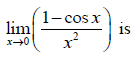Production And Industrial Engineering - PI 2012 GATE Paper (Practice Test) - Question 12

For the spherical surface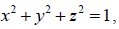the unit outward normal vector at the point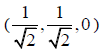is given by

Production And Industrial Engineering - PI 2012 GATE Paper (Practice Test) - Question 13

Consider the function f (x) = |x| in the interval -1 <<1. At the point x = 0, f(x) is

Production And Industrial Engineering - PI 2012 GATE Paper (Practice Test) - Question 14

At x = 0, the function f(x) = x3 + 1 has

Production And Industrial Engineering - PI 2012 GATE Paper (Practice Test) - Question 15

The area enclosed between the straight line y = x and the parabola y = x2 in the x-y plane is

Production And Industrial Engineering - PI 2012 GATE Paper (Practice Test) - Question 16

For a long slender column of uniform cross section, the ratio of critical buckling load for the case with both ends clamped to the case with both ends hinged is

Production And Industrial Engineering - PI 2012 GATE Paper (Practice Test) - Question 17

A thin walled spherical shell is subjected to an internal pressure. If the radius of the shell is increased by 1% and the thickness is reduced by 1%, with the internal pressure remaining the same, the percentage change in the circumferential (hoop) stress is

Production And Industrial Engineering - PI 2012 GATE Paper (Practice Test) - Question 18

A cantilever beam of length L is subjected to a moment M at the free end. The moment of inertia of the beam cross section about the neutral axis is I and the Young’s modulus is E. The magnitude of the maximum deflection is

Production And Industrial Engineering - PI 2012 GATE Paper (Practice Test) - Question 19

A circular solid disc of uniform thickness 20 mm, radius 200 mm and mass 20 kg, is used as a flywheel. If it rotates at 600 rpm, the kinetic energy of the flywheel, in Joules is

Production And Industrial Engineering - PI 2012 GATE Paper (Practice Test) - Question 20

In the mechanism given below, if the angular velocity of the eccentric circular disc is 1 rad/s, the angular velocity (rad/s) of the follower link for the instant shown in the figure is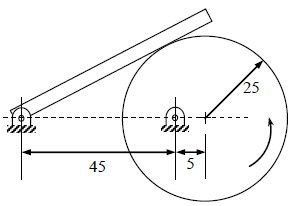Note: All dimensions are in mm.

Production And Industrial Engineering - PI 2012 GATE Paper (Practice Test) - Question 21

Steam enters an adiabatic turbine operating at steady state with an enthalpy of 3251.0 kJ/kg and leaves as a saturated mixture at 15 kPa with quality (dryness fraction) 0.9. The enthalpies of the saturated liquid and vapor at 15 kPa are hf = 225.94 kJ/kg and hg = 2598.3 kJ/kg respectively. The mass flow rate of steam is 10 kg/s. Kinetic and potential energy changes are negligible. The power output of the turbine in MW is

Production And Industrial Engineering - PI 2012 GATE Paper (Practice Test) - Question 22

A n ideal gas of mass m and temperature T1 undergoes a reversible isothermal process from an initial pressure P1 to final pressure P2. The heat loss during the process is Q. The entropy change ΔS of the gas is

Production And Industrial Engineering - PI 2012 GATE Paper (Practice Test) - Question 23

F or an opaque surface, the absorptivity (α ) , transmissivity (τ) and reflectivity (ρ) are related by the equation:

Production And Industrial Engineering - PI 2012 GATE Paper (Practice Test) - Question 24

W hich one of the following configurations has the highest fin effectiveness?

Production And Industrial Engineering - PI 2012 GATE Paper (Practice Test) - Question 25

O il flows through a 200 mm diameter horizontal cast iron pipe (friction factor, f = 0.0225) of length 500 m. The volumetric flow rate is 0.2 m3/s. The head loss (in m) due to friction is (assume g = 9.81 m/s2)

Production And Industrial Engineering - PI 2012 GATE Paper (Practice Test) - Question 26

A solid cylinder of diameter 100 mm and height 50 mm is forged between two frictionless flat dies to a height of 25 mm. The percentage change in diameter is

Production And Industrial Engineering - PI 2012 GATE Paper (Practice Test) - Question 27

In an interchangeable assembly, shafts of size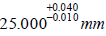mm mate with holes of size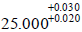mm. The maximum interference (in microns) in the assembly is

Production And Industrial Engineering - PI 2012 GATE Paper (Practice Test) - Question 28

A CNC vertical milling machine has to cut a straight slot of 10 mm width and 2 mm depth by a cutter of 10 mm diameter between points (0, 0) and (100, 100) on the XY plane (dimensions in mm). The feed rate used for milling is 50 mm/min. Milling time for the slot (in seconds) is

Production And Industrial Engineering - PI 2012 GATE Paper (Practice Test) - Question 29

Match the following metal forming processes with their associated stresses in the workpiece.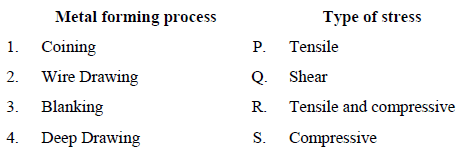Production And Industrial Engineering - PI 2012 GATE Paper (Practice Test) - Question 30

During normalizing process of steel, the specimen is heated

Production And Industrial Engineering - PI 2012 GATE Paper (Practice Test) - Question 31

In abrasive jet machining, as the distance between the nozzle tip and the work surface increases, the material removal rate

Production And Industrial Engineering - PI 2012 GATE Paper (Practice Test) - Question 32

Which one of the following is NOT a decision taken during the aggregate production planning stage?

Production And Industrial Engineering - PI 2012 GATE Paper (Practice Test) - Question 33

Which one of the following is NOT associated with the process of new product development?

Production And Industrial Engineering - PI 2012 GATE Paper (Practice Test) - Question 34

A process needs to be standardized for method and time. Which one of the following represents the sequence of work study experiments?

Production And Industrial Engineering - PI 2012 GATE Paper (Practice Test) - Question 35

Reduction in the variability of manufactured product characteristics will definitely result in observations close to

Production And Industrial Engineering - PI 2012 GATE Paper (Practice Test) - Question 36

Q. 36 - Q. 65 carry two marks each.

Q.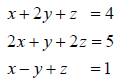The system of algebraic equations given above has

Production And Industrial Engineering - PI 2012 GATE Paper (Practice Test) - Question 37

For the matrix A =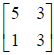, ONE of the normalized eigen vectors is given as

Production And Industrial Engineering - PI 2012 GATE Paper (Practice Test) - Question 38

Consider the differential equation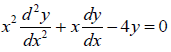with the boundary conditions of
y(0) = 0 and y(1) = 1. The complete solution of the differential equation is

Production And Industrial Engineering - PI 2012 GATE Paper (Practice Test) - Question 39

The inverse Laplace transform of the function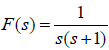is given by

Production And Industrial Engineering - PI 2012 GATE Paper (Practice Test) - Question 40

A box contains 4 red balls and 6 black balls. Three balls are selected randomly from the box one after another, without replacement. The probability that the selected set contains one red ball and two black balls is

Production And Industrial Engineering - PI 2012 GATE Paper (Practice Test) - Question 41

T he state of stress at a point under plane stress condition is

σxx = 40 MPa, σyy = 100 MPa and τxy = 40 MPa.

The radius of the Mohr’s circle representing the given state of stress in MPa is

Production And Industrial Engineering - PI 2012 GATE Paper (Practice Test) - Question 42

A force of 400 N is applied to the brake drum of 0.5 m diameter in a band-brake system as shown in the figure, where the wrapping angle is 180°. If the coefficient of friction between the drum and the band is 0.25, the braking torque applied, in N.m is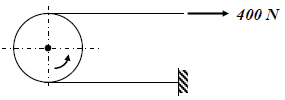Production And Industrial Engineering - PI 2012 GATE Paper (Practice Test) - Question 43

Water (Cp = 4.18 kJ/kg.K) at 80°C enters a counterflow heat exchanger with a mass flow rate of 0.5 kg/s. Air (Cp = 1 kJ/kg.K) enters at 30°C with a mass flow rate of 2.09 kg/s. If the effectiveness of the heat exchanger is 0.8, the LMTD (in °C) is

Production And Industrial Engineering - PI 2012 GATE Paper (Practice Test) - Question 44

C onsider two infinitely long thin concentric tubes of circular cross section as shown in the figure. If D1 and D2 are the diameters of the inner and outer tubes respectively, then the view factor F22 is given by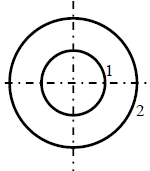Production And Industrial Engineering - PI 2012 GATE Paper (Practice Test) - Question 45

A large tank with a nozzle attached contains three immiscible, inviscid fluids as shown. Assuming that the changes in h1, h2 and h3 are negligible, the instantaneous discharge velocity is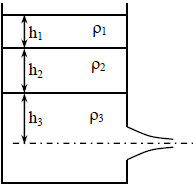Production And Industrial Engineering - PI 2012 GATE Paper (Practice Test) - Question 46

In a DC arc welding operation, the voltage-arc length characteristic was obtained as Varc = 20 + 5l where the arc length l was varied between 5 mm and 7 mm. Here Varc denotes the arc voltage in Volts. The arc current was varied from 400 A to 500 A. Assuming linear power source characteristic, the open circuit voltage and the short circuit current for the welding operation are

Production And Industrial Engineering - PI 2012 GATE Paper (Practice Test) - Question 47

In a single pass rolling process using 410 mm diameter steel rollers, a strip of width 140 mm and thickness 8 mm undergoes 10 % reduction of thickness. The angle of bite in radians is

Production And Industrial Engineering - PI 2012 GATE Paper (Practice Test) - Question 48

A mould having dimensions 100 mm × 90 mm × 20 mm is filled with molten metal through a gate as shown in the figure. For height h and cross-sectional area A, the mould filling time is t1. The height is now quadrupled and the cross-sectional area is halved. The corresponding filling time is t2. The ratio t2/t1 is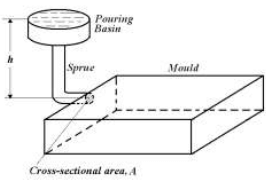Production And Industrial Engineering - PI 2012 GATE Paper (Practice Test) - Question 49

A thin square plate ABCD with side of unit length is kept in the X-Y plane as shown in the figure. The plate is first rotated by 30° in the anti clockwise direction about the Z-axis with A fixed at the origin. The plate is then rotated by 90° in the anti clockwise direction about the X-axis with A fixed at the origin. The final co-ordinates of C are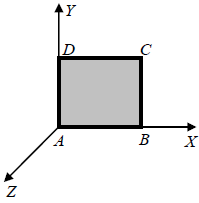Production And Industrial Engineering - PI 2012 GATE Paper (Practice Test) - Question 50

A sine bar has a length of 250 mm. Each roller has a diameter of 20 mm. During taper angle measurement of a component, the height from the surface plate to the centre of a roller is 100 mm. The calculated taper angle (in degrees) is

Production And Industrial Engineering - PI 2012 GATE Paper (Practice Test) - Question 51

M atch the following plastic products with their most appropriate materials.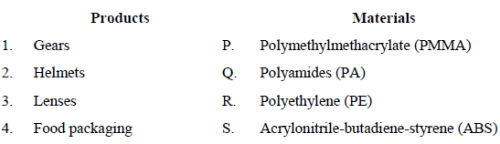Production And Industrial Engineering - PI 2012 GATE Paper (Practice Test) - Question 52

In a shaping process, the number of double strokes per minute is 30 and the quick return ratio is 0.6. If the length of the stroke is 250 mm, the average cutting velocity in m/min is

Production And Industrial Engineering - PI 2012 GATE Paper (Practice Test) - Question 53

For a linear programming problem, the set of constraints x+y ≤ 2, 3x+5y ≥ 15, x ≥ 0 and y ≥ 0 leads to

Production And Industrial Engineering - PI 2012 GATE Paper (Practice Test) - Question 54

A milk vendor incurs an overstocking cost of Rs. 2 per litre and a shortage cost of Rs. 0.5 per litre.
The demand is uniformly distributed between 1 litre and 6 litres. Using the Newsvendor Model, the
maximum quantity of milk in litre(s) the vendor should order is

Production And Industrial Engineering - PI 2012 GATE Paper (Practice Test) - Question 55

The specification limits for the weight of a product are 13.1 kg and 15 kg. If the process has a variance of weight 0.05 kg2, then the process capability index is

Production And Industrial Engineering - PI 2012 GATE Paper (Practice Test) - Question 56

On an average, there are 30 customers in a queue. If the arrival rate of customers into the system is 16 customers per hour and on average 32 customers leave the system per hour, then the average number of customers in the system is

Production And Industrial Engineering - PI 2012 GATE Paper (Practice Test) - Question 57

Data for four jobs that need to be processed on a single machine are given below.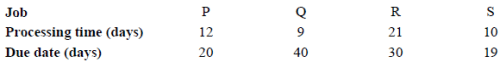All the four jobs are available at time zero. If the jobs are scheduled using the Earliest Due Date (EDD) algorithm, then the job with maximum tardiness is

Production And Industrial Engineering - PI 2012 GATE Paper (Practice Test) - Question 58

Common Data Questions

Common Data for Questions 58 and 59:

Two steel truss members, AC and BC, each having cross sectional area of 100 mm2, are subjected to a horizontal force F as shown in figure. All the joints are hinged.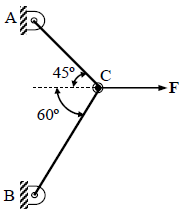Q. The maximum force F in kN that can be applied at C such that the axial stress in any of the truss members DOES NOT exceed 100 MPa is

Production And Industrial Engineering - PI 2012 GATE Paper (Practice Test) - Question 59

Common Data Questions

Common Data for Questions 58 and 59:

Two steel truss members, AC and BC, each having cross sectional area of 100 mm2, are subjected to a horizontal force F as shown in figure. All the joints are hinged..

Q. If F = 1 kN, the magnitude of the vertical reaction force developed at the point B in kN is

Production And Industrial Engineering - PI 2012 GATE Paper (Practice Test) - Question 60

Common Data for Questions 50 and 51:

Data for a plain milling operation are given below.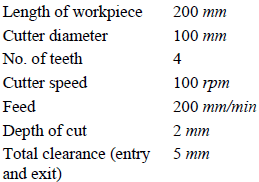Q. Mean undeformed chip thickness (in microns) is

Production And Industrial Engineering - PI 2012 GATE Paper (Practice Test) - Question 61

Common Data for Questions 50 and 51:

Data for a plain milling operation are given below.Q. M achining time for a single pass (in seconds) is

Production And Industrial Engineering - PI 2012 GATE Paper (Practice Test) - Question 62

In an EDM process using RC relaxation circuit, a 12 mm diameter through hole is made in a steel plate of
50 mm thickness using a graphite tool and kerosene as dielectric. Assume discharge time to be negligible.
Machining is carried out under the following conditions:

Resistance 40 Ω
Capacitance 20 μF
Supply voltage 220 V
Discharge voltage 110 V

Q. The time for one cycle, in milliseconds, is

Production And Industrial Engineering - PI 2012 GATE Paper (Practice Test) - Question 63

In an EDM process using RC relaxation circuit, a 12 mm diameter through hole is made in a steel plate of
50 mm thickness using a graphite tool and kerosene as dielectric. Assume discharge time to be negligible.
Machining is carried out under the following conditions:

Resistance 40 Ω
Capacitance 20 μF
Supply voltage 220 V
Discharge voltage 110 V

Q. Average power input (in kW) is

Production And Industrial Engineering - PI 2012 GATE Paper (Practice Test) - Question 64

In a particular year, an organization earns cash revenues of Rs. 2,00,000. Total material and labour expenses are Rs. 1,09,000. The depreciation claimed on the equipment is Rs. 25,000. The tax rate is 20%.

Q. The profit after tax (PAT) is

Production And Industrial Engineering - PI 2012 GATE Paper (Practice Test) - Question 65

In a particular year, an organization earns cash revenues of Rs. 2,00,000. Total material and labour expenses are Rs. 1,09,000. The depreciation claimed on the equipment is Rs. 25,000. The tax rate is 20%.

Q. The net cash flow is

## GATE Past Year Papers for Practice (All Branches)

407 docs|127 tests
Information about Production And Industrial Engineering - PI 2012 GATE Paper (Practice Test) Page
In this test you can find the Exam questions for Production And Industrial Engineering - PI 2012 GATE Paper (Practice Test) solved & explained in the simplest way possible. Besides giving Questions and answers for Production And Industrial Engineering - PI 2012 GATE Paper (Practice Test), EduRev gives you an ample number of Online tests for practice

## GATE Past Year Papers for Practice (All Branches)

407 docs|127 tests(Scan QR code)